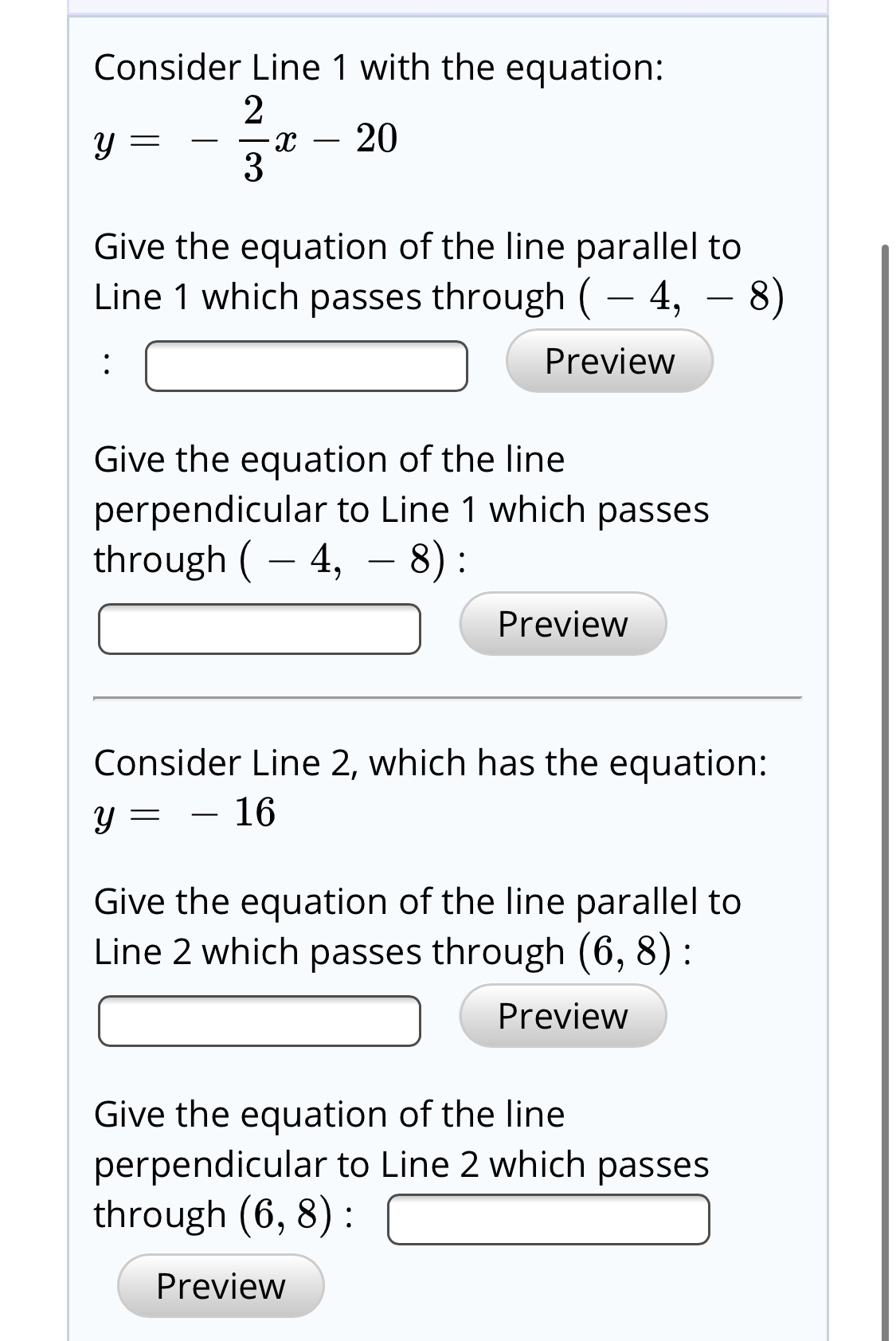# Consider Line 1 with the equation:203Give the equation of the line parallel toLine 1 which passes through (– 4, – 8)Preview:Give the equation of the lineperpendicular to Line 1 which passesthrough ( – 4,- 8):PreviewConsider Line 2, which has the equation:16Give the equation of the line parallel toLine 2 which passes through (6, 8) :PreviewGive the equation of the lineperpendicular to Line 2 which passesthrough (6, 8) :Preview

Question
43 viewshelp_outlineImage TranscriptioncloseConsider Line 1 with the equation: 20 3 Give the equation of the line parallel to Line 1 which passes through (– 4, – 8) Preview : Give the equation of the line perpendicular to Line 1 which passes through ( – 4, - 8): Preview Consider Line 2, which has the equation: 16 Give the equation of the line parallel to Line 2 which passes through (6, 8) : Preview Give the equation of the line perpendicular to Line 2 which passes through (6, 8) : Preview fullscreen
check_circle

Step 1

Since you have asked multiple questions, we will solve the first question for you. If you want any specific question to be solved then please repost the one you’d like to get answered.

Step 2

Consider Line 1 with the equation:

Step 3

Use the slope to find t...

### Want to see the full answer?

See Solution

#### Want to see this answer and more?

Solutions are written by subject experts who are available 24/7. Questions are typically answered within 1 hour.*

See Solution
*Response times may vary by subject and question.
Tagged in